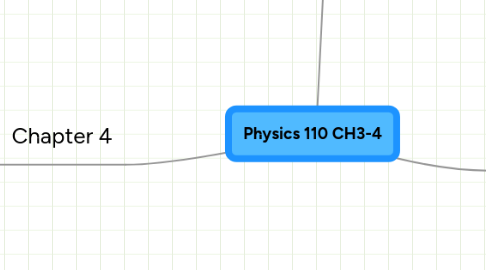# Physics 110 CH3-4

Jetzt loslegen. Gratis!Physics 110 CH3-4 von## 1. Chapter 3

### 1.1. Position

1.1.1. Origin

1.1.1.1. A reference point

1.1.2. We give distance from the origin and the direction

1.1.3. Vector

1.1.3.1. Distance

1.1.3.2. Direction

1.1.4. Graphically

1.1.4.1. The position vector can be drawn as an arrow starting at the origin and ending with the arrowhead on the object

1.1.4.2. When drawing more than one position vector, choose a scale so the length of the vector arrow is proportional to the actual distance between the object and the origin

### 1.2. Displacement

1.2.1. Change of the position vector

1.2.1.1. The final position vector minus the initial position vector

1.2.1.2. Vector subtraction

1.2.1.2.1. To subract a vector is to add its opposite

1.2.2. Any direction in the horizontal plane can be specified by giving an angle with respect to the directions of the compass

### 1.3. Velocity

1.3.1. Vector

1.3.1.1. Magnitude

1.3.1.1.1. Speed

1.3.1.2. Direction

1.3.1.2.1. Direction of motion

1.3.2. Velocity depends on both the displacement and the time interval

1.3.3. Average Velocity

1.3.3.1. Product of...

1.3.3.1.1. Vector

1.3.3.1.2. Displacement

1.3.3.1.3. A positive scalar

1.3.3.1.4. The inverse of the time interval

1.3.3.2. Slope of chord

1.3.3.2.1. rise / run

1.3.3.2.2. Chord- the slope of a line connecting two points

1.3.4. Instantaneous Velocity

1.3.4.1. A vector quantity whose magnitude is the speed and whose direction is the direction of motion

1.3.4.2. Can be used to calculate the displacement of the object during a very short time interval

1.3.5. Rate of change of position

### 1.4. Newton's Second Law of Motion

1.4.1. The rate of change of the velocity is proportional to the net force and inversely proportional to the mass of the object

1.4.2. F = Ma

1.4.2.1. F is net force, M is mass, a is acceleration

1.4.2.1.1. The rate of change of the velocity is acceleration

1.4.3. When the net force is constant, the acceleration is also constant

1.4.4. SI UNITS

1.4.4.1. 1N= 1 kg*(m/s)/s

1.4.4.2. Acceleration

1.4.4.2.1. (m/s)/s

1.4.4.2.2. the rate of change of velocity

1.4.4.2.3. is inversely proportional to the object's mass

1.4.4.3. Force

1.4.4.3.1. Newton

1.4.5. Instantaneous acceleration

1.4.5.1. calculate the average acceleration during a very short time interval

1.4.6. Average acceleration

1.4.7. Problem solving strategy

1.4.7.1. Decide what objects will have Newton's 2nd law applied to them

1.4.7.2. identify all the external forces acting on that object

1.4.7.3. draw a free-body diagram to show all the forces acting on the object

1.4.7.4. Choose a coordinate system

1.4.7.5. Find the net force by adding the forces as vectors

1.4.7.6. relate the acceleration to the change in the velocity vector during a time interval of interest

### 1.5. Mass

1.5.1. Measure of object's inertia

1.5.1.1. the amount of resistance of changes in velocity

## 2. Chapter 4

### 2.1. Kinematics

2.1.1. Study of linear motion

2.1.1.1. Break the complicated motion into intervals

2.1.2. Types of motion

2.1.2.1. Non-constant Acceleration

2.1.2.2. Constant Acceleration

2.1.2.2.1. a = 0 (m/s)/s

2.1.2.2.2. a = + or - 9.8 (m/s)/s

2.1.2.2.3. a = constant

2.1.2.2.4. Special case

2.1.3. Kinematical Equations of Motion for 1-D

2.1.3.1. v = (vo) + at

2.1.3.2. x = (xo) + (vo)t + 1/2 * a(t*t)

2.1.3.3. v*v = (vo*vo) + 2a (xf - xo)

2.1.4. Problem solving techniques for kinematics

2.1.4.1. Wants / Knowns

2.1.4.2. Draw picture

2.1.4.3. Set coordinate axes and assign symbols to numbers

2.1.4.4. Choose the correct equation(s)

2.1.4.5. Solve for the variable of interest

## 3. Words of the Day

### 3.1. Mash-Up

3.1.1. Combining data from more than one source into a single integrated tool

### 3.2. Red Herring

3.2.1. Something intended to divert attention from the real problem or matter at hand

### 3.3. Panacea

3.3.1. A remedy for all difficulties

3.3.2. Cure-All

### 3.4. Ithaca

3.4.1. Get to Destination

### 3.5. Ne Plus Ultra

3.5.1. The acme, most profound degree

### 3.6. Modus Operandi

3.6.1. Mode of operation, Process

### 3.7. Forestall

3.7.1. To deal with or think of beforehand

3.7.2. anticipate